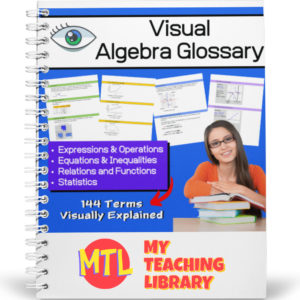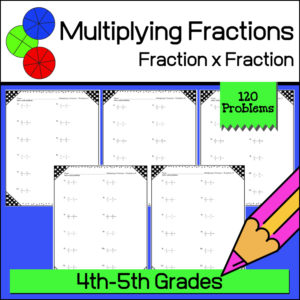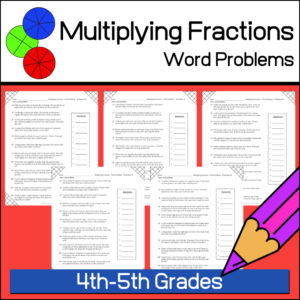Showing 1–20 of 177 results

•\$2.50

Here are 10 Skip Counting Worksheets for students to practice skip counting. Students will practice skip counting both up and down. This resource includes 10 worksheets, each with 10 problems:

• 4 worksheets – counting by twos
• 3 worksheets – counting by fives
• 3 worksheets – counting by tens

Each worksheet also includes a fun Thanksgiving themed maze. Perfect to use in November or really anytime throughout the year!

•\$3.00

Give your students the practice they need with the First Grade Math Skills Workbook. This fun, November themed review resource will give students extra practice in the following areas:

• – Addition facts to 20
• – Addition higher than 20 (no carry)
• – Finding the missing addends
• – Adding doubles to 24
• – Subtraction facts to 20
• – Word problems (Solving and explain through pictures) – addition and subtraction
• – Skip counting by 2 (Filling in the missing numbers)
• – Creating equal addition equations
• Sale!\$7.00 \$5.50

This consumer math bundle will teach students about both simple and compound interest and give them the practice they need to help them master solving word problems!

Includes:

•\$1.50

Students will complete a crossword puzzle by answering compound interest word problems. Also included, an optional number search puzzle which will provide an answer bank to use when completing the crossword puzzle! Answer Keys provided.

Sample problem: How much interest does a \$472 investment earn at 7% compounded annually over eight years?

•\$1.50

Students will complete a crossword puzzle by answering simple interest word problems. Also included, an optional number search puzzle which will provide an answer bank to use when completing the crossword puzzle! Answer Keys provided.

Sample problem: If you put \$5.62 in a saving account that pays 3% for eight years, what is the amount of money you will have at the end of the eight years?

•\$4.00

This consumer math resource has been designed to help teach students and give them the practice they need to master the concepts taught.

The first two pages will explain interest (simple and compound) and give students the formulas they will need to solve the word problems. There are 60 word problems in all. 3 sets of 10 simple interest problems and 3 sets of 10 compound interest problems. Answer keys are provided.

•\$3.50

Give students the practice they need when learning to solve one-step, one-variable equations! This resource provides 7 different worksheets, each with 25 problems for a total of 175 problems. All equations in this set of worksheets use only the properties of addition and subtraction.

•\$15.00

Give your Algebra I and Algebra II students a tool they can really use! This Visual Algebra Glossary will be an invaluable resource giving them explanations, definitions and examples of 144 terms. Easy to follow and understand, students will turn to this resource while learning new concepts, completing work and studying for exams.

There are four color coded sections, each with an term index:

• – Expressions & Operations
• – Equations & Inequalities
• – Relations & Functions
• – Statistics

73 pages

•\$8.00

This 38 page math glossary has been designed for 3rd-5th grades and includes 129 math terms with definitions and 72 illustrations! Terms include those surrounding basic operations, geometry and elementary statistics.

(See description below for a complete list of included terms)

•\$1.50

Help students learn and remember the difference between regular and irregular shapes by displaying this classroom poster!

•\$3.00

In geometry, students need to be able to identify a variety of irregular shapes! Here are 10 worksheets for students to practice identifying the following shapes:

• – pentagon
• – hexagon
• – nonagon
• – heptagon
• – octagon
• – decagon

•\$3.00

This resource is designed to give students the practice the need to become proficient at multiplying decimals. There are three levels, 15 worksheets (16 problems each) and answer keys. That’s 240 problems – EACH WORKSHEET includes a different growth mindset quote!
(See description below for more details)

•\$3.00

This resource is designed to give students the practice the need to become proficient at multiplying decimal numbers. There are three levels, 15 worksheets (20 problems each) and answer keys. That’s 300 problems! Use as practice sheets, homework, or for quizzes and tests.

Also included: Visual poster to help students with ‘where to place’ the decimal point in their answers.

3 Levels:

• Level 1 (5 worksheets)
Tenths multiplied by tenths
• Level 2 (5 worksheets)
Tenths multiplied by hundreds and/or by thousandths
Hundredths multiplied by hundredths and/or by thousandths
• Level 3 (5 worksheets)
Mixed from tenths/hundredths/thousandths multiplied by tenths/hundredths/thousandths
•\$2.00

6 colorful classroom posters showing 3D shapes

Shapes include: cone, pyramid, cube, prism, cylinder, sphere

•\$3.00

Give your students the practice they need to develop visual discrimination and basic math skills with 42 engaging worksheets! Students will interactively identify big or small objects by coloring pictures, circling or tracing the words ‘big’ or ‘small’, cutting, pasting and sorting (classifying) pictures. This type of engagement will also allow your students to use and develop fine motor skills!

•\$3.00

Give your students the practice and reinforcement they need as they are learning to multiply fractions with fractions! This resource includes 120 problems (10 worksheets with 12 problems each) and answer keys.

•\$3.00

Help students develop their math word problem skills and practice solving multiplying fractions all at the same time! This resources provides ten (10) ready to use, print and go worksheets each with 12 word problems for a total of 120 problems (answer keys provided).

•\$2.50

Give your students the practice and reinforcement they need as they are learning to multiply fractions by whole numbers! This resource includes 100 problems (5 worksheets with 20 problems each) and answer keys.

•\$3.00

Help your students understand and remember the basis of multiplying fractions using a visual model method! This product, Multiplying Fractions with Visual Models #2, gives you 110 problems in which students will multiply a fraction with a another fraction. For each problem students will be asked to color in given visual fraction models to find the answer before giving the answer.

Product includes 10 worksheets (11 questions each) = 110 questions + answer keys.

• Sale!\$9.00 \$7.00

I’ve bundled 3 of my products for students working with decimals! Resource #1 will help students understand equivalent fractions, decimals and percents. Resource #2 will have students working with decimal notation, location on a number line, digit value and relative value in relation to half. Resource #3 will give students a lot of practice adding and subtracting decimals and includes 100 word problems!

There are over 700 problems included in this bundle and a lot of work with visual models. Answer Keys are provided.